# HSSlive: Plus One & Plus Two Notes & Solutions for Kerala State Board

## BSEB Class 10 Maths Chapter 6 Triangles Ex 6.6 Textbook Solutions PDF: Download Bihar Board STD 10th Maths Chapter 6 Triangles Ex 6.6 Book AnswersBSEB Class 10 Maths Chapter 6 Triangles Ex 6.6 Textbook Solutions PDF: Download Bihar Board STD 10th Maths Chapter 6 Triangles Ex 6.6 Book Answers

BSEB Class 10th Maths Chapter 6 Triangles Ex 6.6 Textbooks Solutions and answers for students are now available in pdf format. Bihar Board Class 10th Maths Chapter 6 Triangles Ex 6.6 Book answers and solutions are one of the most important study materials for any student. The Bihar Board Class 10th Maths Chapter 6 Triangles Ex 6.6 books are published by the Bihar Board Publishers. These Bihar Board Class 10th Maths Chapter 6 Triangles Ex 6.6 textbooks are prepared by a group of expert faculty members. Students can download these BSEB STD 10th Maths Chapter 6 Triangles Ex 6.6 book solutions pdf online from this page.

## Bihar Board Class 10th Maths Chapter 6 Triangles Ex 6.6 Books Solutions

 Board BSEB Materials Textbook Solutions/Guide Format DOC/PDF Class 10th Subject Maths Chapter 6 Triangles Ex 6.6 Chapters All Provider Hsslive

## How to download Bihar Board Class 10th Maths Chapter 6 Triangles Ex 6.6 Textbook Solutions Answers PDF Online?

2. Click on the Bihar Board Class 10th Maths Chapter 6 Triangles Ex 6.6 Answers.
3. Look for your Bihar Board STD 10th Maths Chapter 6 Triangles Ex 6.6 Textbooks PDF.
4. Now download or read the Bihar Board Class 10th Maths Chapter 6 Triangles Ex 6.6 Textbook Solutions for PDF Free.

Find below the list of all BSEB Class 10th Maths Chapter 6 Triangles Ex 6.6 Textbook Solutions for PDF’s for you to download and prepare for the upcoming exams:

## BSEB Bihar Board Class 10th Maths Solutions Chapter 6 Triangles Ex 6.6

Question 1.
In the figure, PS is the bisector of ∠QPR of ∆ PQR. Prove that 𝑄𝑆𝑆𝑅 = 𝑃𝑄𝑃𝑅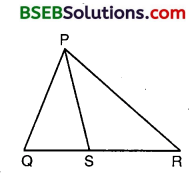Solution:
Given : PQR is a triangle and PS is the bisector of ∠QPR meeting QR at S.
∴ ∠QPS = ∠SPR
To Prove : 𝑄𝑆𝑆𝑅 = 𝑃𝑄𝑃𝑅
Construction : Draw RT || SP to cut QP produced at T.
Proof :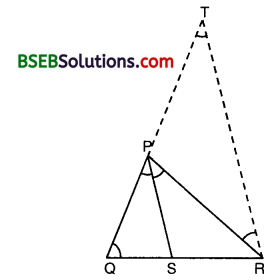Since PS || TR and PR cuts them, hence we have :
∠SPR = ∠PRT … (1) [Alternate ∠S]
and ∠QPS = ∠PTR … (2) [Corresponding ∠S]
But, ∠QPS = ∠SPR [Given]
∴ ∠PRT = ∠PTR [From (1) and (2)]
So, PT = PR … (3) [∵ Sides opp. to equal ∠S are equal]
Now, in ∆ QRT, we have
SP || RT [By Construction]
∴ 𝑄𝑆𝑆𝑅 = 𝑃𝑄𝑃𝑇 [By Basic Proportionality Theorem]
or 𝑄𝑆𝑆𝑅 = 𝑃𝑄𝑃𝑅 [From (3)]

Question 2.
In the figure, D is a point on hypotenuse AC of ∆ ABC, BD ⊥ AC, DM ⊥ BC and DN ⊥ AB. Prove that(i) DM² = DN.MC
(ii) DN² = DM.AN
Solution:
We have : AB ⊥ BC and DM ⊥ BC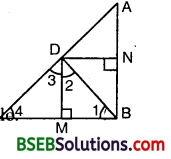So, AB || DM
Similarly, we have :
BC ⊥ AB and DN ⊥ AB.
So, CB || DN
Hence, quadrilateral BMDN is a rectangle.
BM = ND

(ii) In ∆ BMD, we have :
∠1 + ∠BMD + ∠2 = 180°
or ∠1 + 90° + ∠2 = 180° or ∠1 + ∠2 = 90°
Similarly, in ∆ DMC, we have :
∠3 +∠4 = 90°
Since BD ⊥ AC, therefore
∠2 + ∠3 = 90°
Now, ∠1 + ∠2 = 90° and ∠2 + ∠3 = 90°
∴ ∠1 + ∠2 = ∠2 + ∠3
So, ∠1 = ∠3
Also, ∠3 +∠4 = 90° and ∠2 + ∠3 = 90°
∴ ∠3 +∠4 = ∠2 + ∠3 So, ∠2 =∠4
Thus, in As BMD and DMC, we have :
∠1 = ∠3 and ∠2 =∠4
∴ By AA criterion of similarity, we have :
∆ BMD ~ ∆ DMC
So, 𝐵𝑀𝐷𝑀 = 𝑀𝐷𝑀𝐶
or 𝐷𝑁𝐷𝑀 = 𝐷𝑀𝑀𝐶 [∵BM = ND]
so, DM² = DN.MC

(ii) Proceeding as in (i), we can prove that
∆ BMD ~ ∆ DMC
So, 𝐵𝑁𝐷𝑁 = 𝑁𝐷𝑁𝐴
or 𝐷𝑀𝐷𝑁 = 𝐷𝑁𝐴𝑁 [∵BN = DM]
so, DN² = DM.AN

Question 3.
In the figure, ABC is a triangle in which ∠ABC >90° and AD ⊥ CB produced. Prove that AC² = AB² + BC² + 2BC.BD.Solution:
Given : ABC is a triangle in which ∠ABC > 90° and AD ⊥ CB produced.
To Prove : AC² = AB² + BC² + 2BC.BD.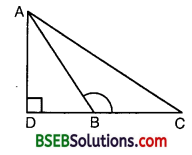Proof : Since ∆ ADB is a right triangle, right angled at D, therefore, by Pythagoras theorem, we have :
AB² = AD² + DB² … (1)
Again, ∆ ADC is a right triangle, right angled at D.
Therefore, by Pythagoras theorem, we have
or AC² =AD² + (DB + BC)2
or AC² =AD² + DB²+ BC² + 2DB.BC
or AC² = (AD² + DB²) + BC² + 2BC.BD
or AC² = AB² +BC² + 2BC.BD [Using (1)]
which proves the required result.

Question 4.
In the figure, ABC is a triangle in which ∠ABC < 90° and AD ⊥ BC. Prove that
AC² = AB² + BC² – 2BC.BD.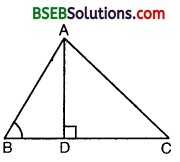Solution: Given : ABC is a triangle in which ∠ABC < 90° and AD ⊥ BC.
To Prove : AC² = AB² + BC² – 2BC . BD.
Proof : Since ∆ ADB is a right triangle, right angled at D, therefore, by Pythagoras theorem, we have :
AB² = AD² + BD² … (1)
Again, ∆ ADC is a right triangle, right angled at D. Therefore, by Pythagoras theorem, we have :
or AC² = AD² + (BC – BD)²
or AC² = AD² + (BC² + BD² – 2BC.BD)
or AC² = (AD² + BD²) + BC² – 2BC.BD
or AC² = AB² + BC² – 2BC.BD [Using (1)]
which proves the required result.

Question 5.
In the figure, AD is a median of a triangle ABC and AM ⊥ BC. Prove that(i) AC² = AD² + BC . DM + (𝐵𝐶2)²
(ii) AB² = AD² – BC . DM + (𝐵𝐶2)²
(iii) AC² + AB² = 2AD² + 12BC².
Solution:
Since ∠AMD = 90°, therefore ∠ADM < 90° and ∠ADC > 90°.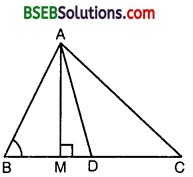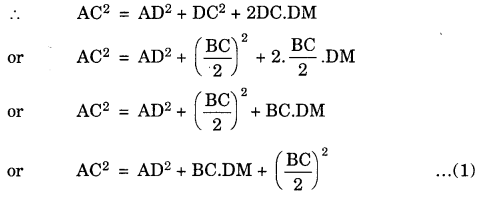(ii) In ∆ ABD, ∠ADB is an acute angle.(iii) From (1) and (2), we get
AB² + AC² = 2AD² + 12BC².

Question 6.
Prove that the sum of the squares of the diagonals of a parallelogram is equal to the sum of the squares of its sides.
Solution:
We know that if AD is a median of ∆ ABC, then AB² + AC² = 2AD² + 12BC²
Since the diagonals of a parallelogram bisect each other, therefore BO and DO are medians of ∆s ABC and ADC respectively.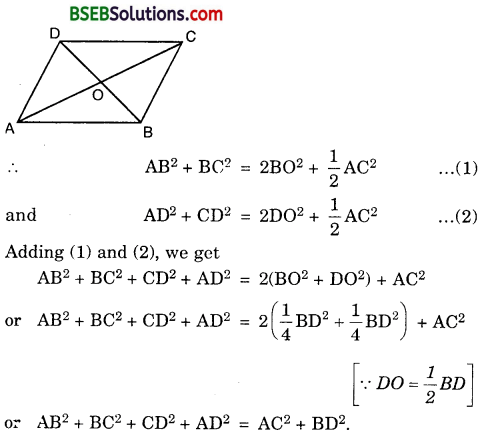Question 7.
In the figure, two chords AB and CD intersect each other at the point P. Prove that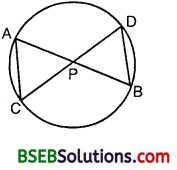(i) ∆ APC ~ ∆ DPB
(ii) AP.PB = CP.DP
Solution:
(i) In ∆s APC and DPB, we have
∠APC = ∠DPB
∠CAP = ∠BDP [Angles in the same segment of a circle are equal]
∴ By AA criterion of similarity, we have :
∆ APC ~ ∆ DPB.

(ii) Since ∆ APC ~ ∆ DPB, therefore
∴ 𝐴𝑃𝐷𝑃 = 𝐶𝑃𝑃𝐵 or AP.PB = CP.DP

Question 8.
In the figure, two chords AB and CD of a circle intersect each other at the point P (when produced) outside the circle. Prove that(i) ∆ PAC ~ ∆ PDB
(ii) PA.PB = PC.PD
Solution:
(i) In ∆s PAC and PDB, we have :
∠APC = ∠BPD [Common]
∠PAC = ∠PDB [∵∠BAC = 180° – ∠PAC and ∠PDB = ∠CDB = 180° – ∠BAC = 180° – (180° – ∠PAC) = ∠PAC]
∴ By AA criterion of similarity, we have
∆ PAC ~ ∆ PDB

(ii) Since ∆ PAC ~ ∆ PDB, therefore
𝑃𝐴𝑃𝐷 = 𝑃𝐶𝑃𝐵 OR PA.PB = PC.PD

Question 9.
In the figure, D is a point on side BC of ∆ ABC such that 𝐵𝐷𝐶𝐷 = 𝐴𝐵𝐴𝐶. Prove that AD is the bisector of ∠BAC.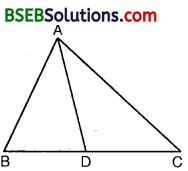Solution:
Given : ABC is a triangle and D is a point on BC such that
𝐵𝐷𝐶𝐷 = 𝐴𝐵𝐴𝐶
To Prove : AD is the bisector of ∠BAC.
Construction : Produce BA to E such that AE = AC. Join CE.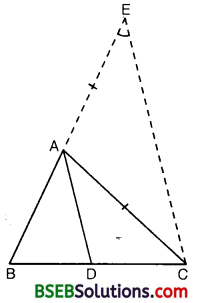Proof : In ∆ AEC, since AE = AC, hence
∠AEC = ∠ACE …(1) [∵ Angles opp. to equal sides of a A are equal]
Now, 𝐵𝐷𝐶𝐷 = 𝐴𝐵𝐴𝐶 [Given]
So, 𝐵𝐷𝐶𝐷 = 𝐴𝐵𝐴𝐸 [∵ AE = AC, construction]
∴ By converse of Basic Proportionality Theorem, we have :
DA || CE
Now, since CA is a transversal, we have :
∠BAD = ∠AEC … (2) [Corresponding ∠S]
and ∠DAC = ∠ACE … (3) [.Alternate angles]
Also, ∠AEC – ∠ACE [From (1)]
Hence, ∠BAD= ∠DAC [From (2) and (3)]

Question 10.
Nazima is fly fishing in a stream. The tip of her fishing rod is 1.8 m above the surface of the water and the fly at the end of the string rests on the water 3.6 m away and 2.4 m from a point directly under the tip of the rod. Assuming that her string (from the tip of her rod to the fly) is taut, how much string does she have out (see figure) ? If she pulls in the string at the rate of 5 cm per second, what will be the horizontal distance of the fly from her after 12 seconds ?Solution:
In fact, we want to find. AC.By Pythagoras Theorem, we have :
AC² – (2.4)² + (1.8)²
or AC² = 5.76 + 3.24
= 9.00
or AC = 3 m
∴ Length of string she have out = 3m.
Length of the string pulled at the rate of 5 cm/sec in 12 seconds
= (5 x 12) cm
= 60 cm = 0.60 m
Remaining string left out
= (3 – 0.6) m
= 2.4 m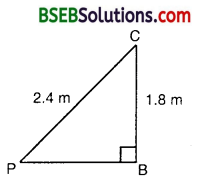In 2nd case, let us find PB.
PB² = PC² – BC²
= (2.4)² – (1.8)²
= 5.76 – 3.24 = 2.52
or PB = 2.52‾‾‾‾√ = 1.59 (nearly)
Hence, the horizontal distance of the fly from Nazima after 12 seconds
= (1.59 + 1.2) m
= 2.79 m (nearly).

## Bihar Board Class 10th Maths Chapter 6 Triangles Ex 6.6 Textbooks for Exam Preparations

Bihar Board Class 10th Maths Chapter 6 Triangles Ex 6.6 Textbook Solutions can be of great help in your Bihar Board Class 10th Maths Chapter 6 Triangles Ex 6.6 exam preparation. The BSEB STD 10th Maths Chapter 6 Triangles Ex 6.6 Textbooks study material, used with the English medium textbooks, can help you complete the entire Class 10th Maths Chapter 6 Triangles Ex 6.6 Books State Board syllabus with maximum efficiency.

## FAQs Regarding Bihar Board Class 10th Maths Chapter 6 Triangles Ex 6.6 Textbook Solutions

#### Can we get a Bihar Board Book PDF for all Classes?

Yes you can get Bihar Board Text Book PDF for all classes using the links provided in the above article.

## Important Terms

Bihar Board Class 10th Maths Chapter 6 Triangles Ex 6.6, BSEB Class 10th Maths Chapter 6 Triangles Ex 6.6 Textbooks, Bihar Board Class 10th Maths Chapter 6 Triangles Ex 6.6, Bihar Board Class 10th Maths Chapter 6 Triangles Ex 6.6 Textbook solutions, BSEB Class 10th Maths Chapter 6 Triangles Ex 6.6 Textbooks Solutions, Bihar Board STD 10th Maths Chapter 6 Triangles Ex 6.6, BSEB STD 10th Maths Chapter 6 Triangles Ex 6.6 Textbooks, Bihar Board STD 10th Maths Chapter 6 Triangles Ex 6.6, Bihar Board STD 10th Maths Chapter 6 Triangles Ex 6.6 Textbook solutions, BSEB STD 10th Maths Chapter 6 Triangles Ex 6.6 Textbooks Solutions,
Share: